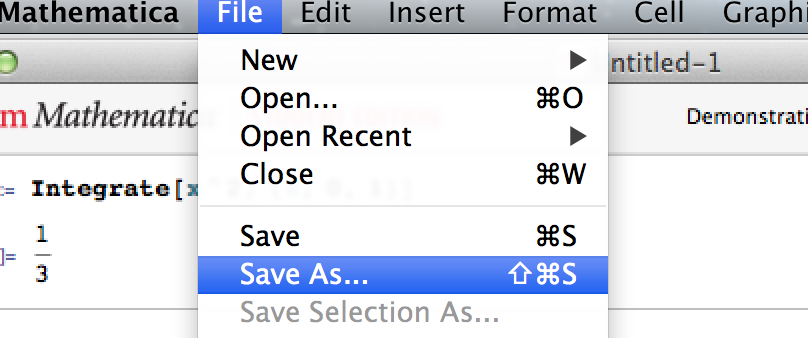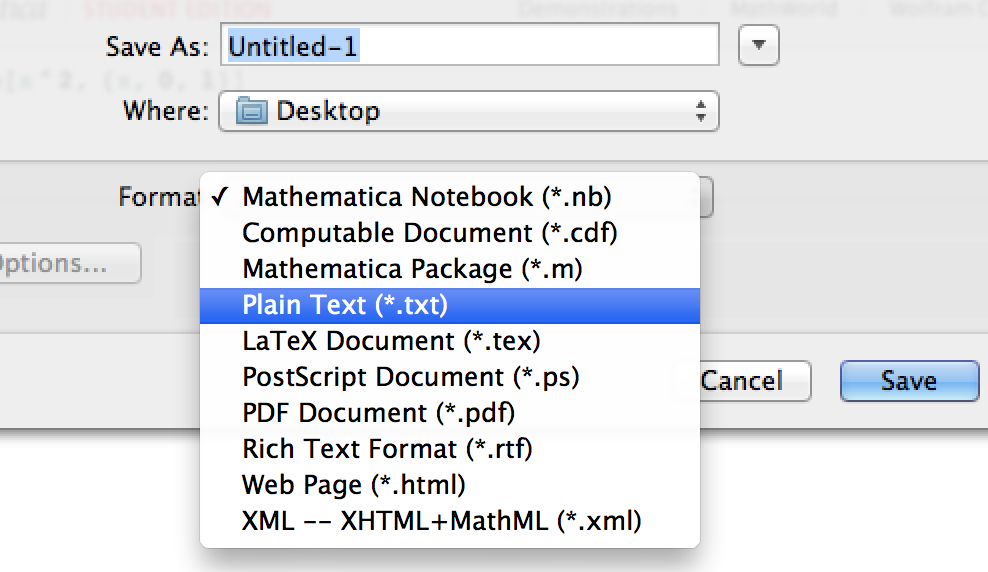# Track Mathematica Source Files with Version Control Systems

Last updated on October 1, 2016

Mathematica is a famous computational software program in science, engineering, etc. However, the source code of Mathematica is usually stored in the format of Mathematica Notebook, which is hard to track with a Version Control System, such as git, mercurial, etc. Here I’m providing a solution to solve this problem.

For example, for a Mathematica notebook with the following simple lines:

``````In:= Integrate[x^2, {x, 0, 1}]
Out= 1/3``````

The contents in this file could be as long as below (You can inspect the contents of the notebook by opening it with a text editor):

Then how should we track a Mathematica source file if it’s a mess like this? The solution is simply: use plain text files to track.

First, edit your Mathematica Notebook files to do integrals, solve equations, etc. as you usually do. After that, click on Save As… under the File menu:Then choose the format as Plain Text (*.txt):OK, now we have a file with the simple content. If we follow the example above, the contents of the file should be:

``````In:= Integrate[x^2,{x,0,1}]
Out= 1/3``````

You can then track this file with your favorite version control system.

When you want to load this file, first, click on Open… under File menu. Then choose the file format as Text File in the Open File Dialog:Finally, choose the plain text file you saved before in the file browser, and enjoy!

Note: I noticed that this method currently doesn’t work for plot. Please let me know if you have a method that works for plot.

This entry was posted in Mathematica, Version Control on by .1.steve
1.Hong Xu